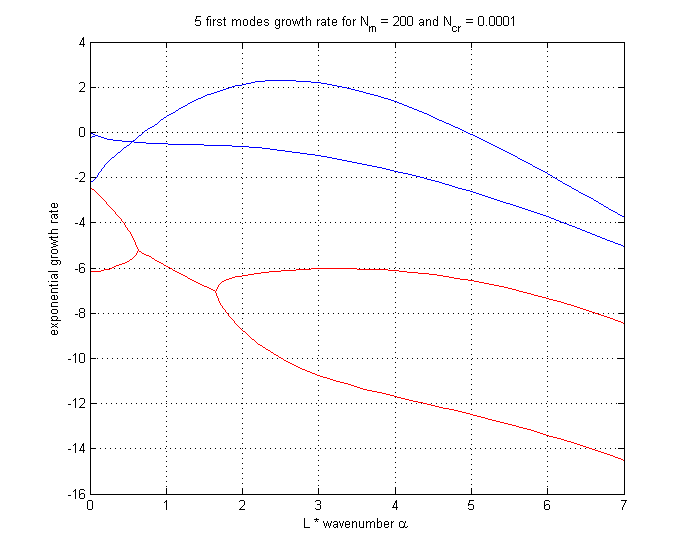# sandbox/easystab/stab2014/rayleigh_benard_marangoni.m

clear all; clf;

# The Rayleigh-Benard-Marangoni convection

This extends the Rayleigh-Benard.m convection. A free surface fluid is heated from below, thus localy changing its density and its surface tension.

This means we have to consider many fluid parameters, listed below. We will study their influence on the stability, depending on the wave numbers considered.

% parameters
N      = 50   ; % number of gridpoints
rho    = 1    ; % fluid density
mu     = 0.001; % fluid viscosity
g      = 1    ; % gravity
sigma0 = 1    ; % initial surface tension
c      = 0.2  ; % surface tension thermal coefficient
d      = 1    ; % thermal dilatation
k      = 0.01 ; % thermal diffusivity
Ty     = -1   ; % vertical gradient of temperature
L      = 0.1  ; % domain height


To run the code you may need Chebychev differentiation matrices, available here

% differentiation matrices
scale=-2/L;
[y,DM] = chebdif(N,2);
D=DM(:,:,1)*scale;
DD=DM(:,:,2)*scale^2;
y=y/scale;
Z=zeros(N,N); I=eye(N);

VAL=[]; % vector used to stock eigenvalues
alphas=[0.001:0.4:20,22:2:70]; % Wavelengths alpha used for calculation

for alpha=alphas % begining the loop on alpha values

% renaming the differentiation matrices
dy=D; dyy=DD;
dx=1i*alpha*I; dxx=-alpha^2*I;
Delta=dxx+dyy;


# System equations

The free surface linearization is explained here

Variables are linearized around initial state : \displaystyle \begin{array}{l} U = u_0+u\\ V=v_0+v\\ T=T_0+y T_y+\theta\\ P=\rho_0 g(L-y)+p \end{array}

Surface tension and density depend on temperature, temperature gradient is supposed small enough to keep thermal coefficients constant :

\displaystyle \begin{array}{l} \rho_{tot} = \rho_0 + \rho (T) \\ \rho(T)=-d \rho_0 \theta \\ \sigma_{tot} = \sigma_0+\sigma(x,T)\\ \sigma(x,T) = -c\sigma_0 \theta \end{array} Incompressibility : \displaystyle u_x+v_y=0 Linearized Navier-stokes around initial state with u_0=v_0=0, with Boussinesq approximation on the y component: \displaystyle \begin{array}{l} \rho_0 u_t = -p_x + \mu \Delta u\\ \rho_0 v_t = -p_y + d \rho_0 g \theta +\mu \Delta v\\ \theta_t+T_y v=k \Delta \theta \end{array}

We can write this system of equations in a matrix form \displaystyle Eq_t=Aq with the matrices \displaystyle q=\left(\begin{array}{c} u \\ v\\ p\\ \theta \\ \eta \\ \sigma \end{array}\right) , \quad A=\left(\begin{array}{cccc} \mu\Delta&0&-\partial_x&0 & 0 & 0 \\ 0&\mu\Delta&-\partial_y&\rho_0 g d & 0 & 0\\ \partial_x&\partial_y&0&0 & 0 & 0\\ 0&-T_y&0&k\Delta &0 & 0\\ 0&I|_L&0&0&0&0\\ 0&0&0& \sigma_0 c I|_L & 0 & 1 \end{array}\right) , \quad E=\left(\begin{array}{cccccc}\rho_0& \\ &\rho_0& \\ & &0& \\ & & &1& \\ & & & &1& \\ & & & & &0\end{array}\right)

% system matrices
A=[mu*Delta, Z, -dx, Z, Z(:,1),Z(:,1);...
Z, mu*Delta, -dy, rho*g*d*I, Z(:,1),Z(:,1);  ...
dx, dy, Z, Z, Z(:,1),Z(:,1);  ...
Z, -Ty*I, Z, k*Delta, Z(:,1),Z(:,1);...
Z(1,:),I(N,:),Z(1,:),Z(1,:),0,0;...
Z(1,:),Z(1,:),Z(1,:),sigma0*c*I(N,:),0,1];
E=blkdiag(rho*I,rho*I,Z,I,1,0);


# Boundary conditions

Non penetration at the bottom : \displaystyle \begin{array}{l} u|_0=0\\ v|_0=0 \end{array} Imposed temperature at the bottom \displaystyle \theta|_0=0 Continuity of heat flux at the interface, assuming the top fluid’s flux is negligible : \displaystyle \theta_y|_L=0

Continuity of stress at interface, in general situation, is expressed with this relation, domain 2 being above domain 1, \mathbf{n} being the normal vector from 2 to 1, and \mathbf{T} the stress tensor : \displaystyle \mathbf{T}_2.\mathbf{n}-\mathbf{T}_1.\mathbf{n} = (\sigma \nabla.\mathbf{n})\mathbf{n}-\nabla \sigma A formal demonstration is given on this page. With \mathbf{T}=-p \mathbf{I}+\mu (\nabla \mathbf{u} + \nabla^t \mathbf{u}) , \mathbf{n}=\left(\begin{array}{l} 0 \\ 1 \end{array}\right) with small perturbations, and expressed in our system, where domain 1 is negligible, this leads to

tangential stress continuity : \displaystyle \mu (u_y+v_x)|_L=\sigma_x normal stress continuity : \displaystyle p|_L=2 \mu v_y|_L + \rho_0 g \eta-\sigma_0 \eta_{xx}

thus the boundary conditions are expressed Cq=0, with the constraint matrix \displaystyle C=\left(\begin{array}{cccccc} I|_0&0&0&0&0&0\\ \mu \partial_y |_L & \mu \partial_x |_L&0&0&0&-i \alpha\\ 0&I|_0&0&0&0&0\\ 0&2 \mu \partial_y |_L&-I|_L&0&\rho_0 g+\sigma_0 \alpha^2&0 \\ 0&0&0&I|_0&0&0\\ 0&0&0&\partial_y |_L&0&0 \end{array}\right)

% boundary conditions

u0=1; uL=N; v0=N+1; vL=2*N ; p0=2*N+1 ; pL=3*N; T0=3*N+1; TL=4*N; eta=4*N+1; %location in vector
loc=[u0,uL,v0,pL,T0,TL];

C=[ I(1,:)     , Z(1,:)       , Z(1,:) , Z(1,:)  , 0                    , 0;...     % u(0)=0
mu*dy(N,:) , mu*dx(N,:)   , Z(1,:) , Z(1,:)  , 0                    ,-1i*alpha; % µ(dv/dx+du/dy)=d(sigma)/dx
Z(1,:)     , I(1,:)       , Z(1,:) , Z(1,:)  , 0                    , 0;...     % v(0)=0
Z(1,:)     , 2*mu*dy(1,:) ,-I(N,:) , Z(1,:)  , rho*g+sigma0*alpha^2 , 0;...     % p(L)=2*mu*dv/dy+rho*g*eta-sigma0*d²(eta)/dx²
Z(1,:)     , Z(1,:)       , Z(1,:) , I(1,:)  , 0                    , 0; ...    % T(0)=0
Z(1,:)     , Z(1,:)       , Z(1,:) , dy(N,:) , 0                    , 0];       % dT(L)/dy=0

A(loc,:)=C;
E(loc,:)=0;


# Results and validation

We compute the eigenmodes of this system and sort them to get the main ones.

To validate the program we use an article of K.A SMITH, published in 1958 in the journal of fluid mechnanics, page 401.

He did an analytic study of this convection, depending on some specific numbers :

Marangoni number N_m=-c \sigma_0 L^2 T_y/{\mu k}

Crispation number N_{cr} = \mu k/{\sigma_0 L}

Group number N_G=\rho_0 L^3 g/\sigma_0

And finally he didn’t consider directly wavenumber \alpha but \alpha L

He summed up his results for low N_G values on this figure. I added red lines to point the values i used : for N_m=200 and N_{cr}=10^{-4} he found that the system was unstable for 0.7<\alpha L<5.

Using the same specific numbers i plot the main eigenvalues, depending on \alpha L.We are above 0 for 0.71<\alpha L<4.95, as Smith was. This validates our model.

% computing eigenmodes
[U,S]=eig(A,E);
s=diag(S);  [t,o]=sort(-real(s)); s=s(o); U=U(:,o);
rem=abs(s)>1000;
s(rem)=[]; U(:,rem)=[];
VAL=[VAL,real(s(1:5))];

end

Nm=-c*sigma0*L^2*Ty/mu/k % Marangoni number
Ncr=mu*k/sigma0/L % Crispation number
Ng=rho*L^3*g/sigma0 % Group number

% Plotting
plot(L*alphas,VAL(1,:),'b',L*alphas,VAL(2,:),'b',L*alphas,VAL(3,:),'b',L*alphas,VAL(4,:),'r',L*alphas,VAL(5,:),'r');
xlabel('L * wavenumber \alpha'); ylabel('exponential growth rate');title(['5 first modes growth rate for N_m = ',num2str(Nm),' and N_{cr} = ',num2str(Ncr)]) ;
grid on;